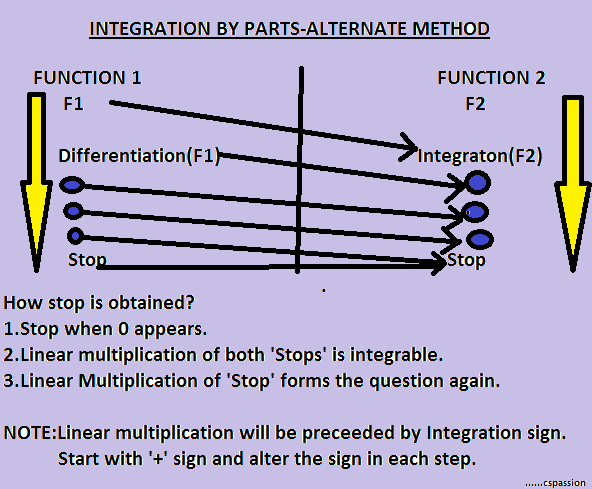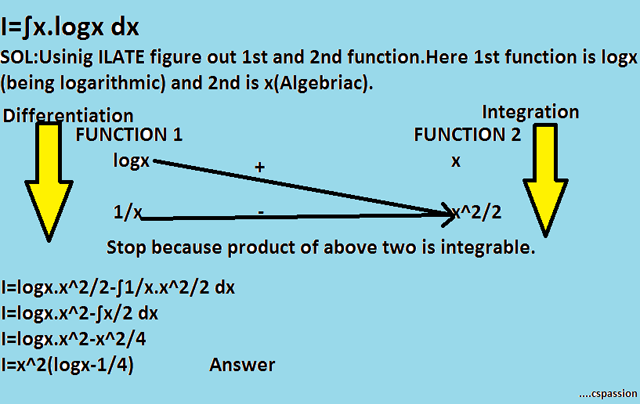## Integration By Parts Easy Method

On 23:12 by Vardan Kumar in    2 comments
Integration By Parts-EASY METHOD

### PRINCIPLE:

The logic behind this type of integration is,it frequently decomposes the integration of product of two functions into an integration problem which is easily solvable.

### ∫uv dx=u∫vdx-∫u'(∫vdx)dx

Here u->Any function u(x)
v->Any function v(x)
u'->Derivative of u(x)

Using this formula may cause confusion and increases the chances of errors.Also we need to memorize this formula.So here we go with....

EASY METHOD:-

Before we begin with the discussion lets just have a quick review of ILATE rule.Using this rule we are gonna characterize our function as Function 1 and Function 2.

I-INVERSE TRIGONOMETRIC FUNCTIONS
L-LOGARITHMIC FUNCTIONS
A-ALGEBRAIC FUNCTIONS
T-TRIGONOMETRIC FUNCTIONS
E-EXPONENTIAL FUNCTIONS

The above order is used in the following way:-

The function which appears above the another function in the above list is named as Function 1 else Function 2.Integration By Parts-Easy Method

### STEPS TO BE FOLLOWED:-

1.Characterize the functions as Function 1 and Function 2.

2.Form two columns with heading Function 1 and function 2 as shown in the above image.

3.Carry out continues differentiation and integration on function 1 and function 2 respectively till 'Stop' is obtained.

4.Draw arrows as shown in the above image.

5.The inclined arrow indicates the simple multiplication.

6.The linear arrow indicate to multiply the two functions and then perform integration on the multiplication obtained.

## Let us illustrate this method with Examples.

### EXAMPLE 1:∫xcosx dx

Solution:Open the below image for solutionIntegration-Example 1

LET US TAKE ANOTHER EXAMPLE.......

EXAMPLE 2: ∫e^x.cosx dx
Solution:Open image below for solutionIntegration-Example 2

LET US REVIEW ONE LAST EXAMPLE.........

EXAMPLE 3:∫x.logx dx
Sol:Refer the image below for solution.....

`Integration-Example 3

NOTE:THE CONSTANT OF INTEGRATION IS NOT INTRODUCED HERE.IT DOES NOT MEAN THAT IT WON'T COME.THE CONSTANY OF INTEGRATION IS ALWAYS INTRODUCED AFTER EVALUATING THE INDEFINITE INTEGRAL.

This was Integration by parts-EASY METHOD.For any queries feel free to comment below or refer the contact form below.

1.1.